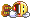#www.vustudents.ning.com

Question 1: 

Match the following to one of the five layers.

a. Route determination

b. Flow control

c. Interface to physical world

e. Packet switching

Question 2: 

If the throughput at the connection between the device and transmission medium is 5 Kbps,how long does it take to send 400,000 bits out of this device?

Question 3: 

Give the Signal level for each line encoding from following.

a. Unipolar

b. NRZ-L

c. NRZ-I

d. RZ

e. AMI

Lectures Covered: This assignment covers Lecture # 08-17DeadlineYour assignment must be uploaded/submitted till Tuesday 03 May, 2011.

Views: 465

### Replies to This Discussion

bhai computer main

1 kilo = 1024

not 1000

Amir Bhaiya Google mai b kilo byte stands for 1024

8 bits = 1 byte

so

5120x8 = 40960 bits

See the attached file for Solution
Attachments:

Time taken= 5*0.38146972

Time taken= 1.9073sec

isko explain be to karain AMIR nai jaisa kiya hai

how??Assignment No. 02 SEMESTER Spring 2011

CS601- Data Communication

Total Marks: 15

Due Date: 03/05/2011

Question 1:                                                                                                                    

Match the following to one of the five layers.

a. Route determination--------------------Network Layer

b. Flow control--------------------Data Link Layer & Transport Layer

c. Interface to physical world------------------------ Application Layer

d. Provide access to the network for the end users--------------- Application Layer

e. Packet switching--------------------- Session Layer

Question 2:                                                                                                                    

If the throughput at the connection between the device and transmission medium is 5 Kbps,

how long does it take to send 400,000 bits out of this device?

Time taken= 5*0.38146972

Time taken= 1.9073sec

Question 3:                                                                                                                    

Give the Signal level for each line encoding from following.

a.Unipolar

This encoding is called unipolar because it uses only one polarity

This encoding is simple and uses only one technique and this encoding is almost obsolete today, however study of this encoding make us familiar with many encoding problems, complexities and techniques.

b. NRZ-L

level of the signal is represented by the data (Bits) it represents.

c. NRZ-I

1 Bit is represented by the inversion of the level.

0 is represented by no change in level

d. RZ

Some time, data contains long strings of 1’s or 0’s, RZ can loose its timing

Signal changes not between the  bits but during each bit

The only problem with RZ encoding is that it requires two signal changes to encode one bit and therefore occupies more BANDWIDTH

e. AMI

(Alternate Mark Inveraion) it the type of bipolar encoding.

By inverting on each occurrence of 1, AMI accomplishes 2 things i.e ;

1-Long sequence of 1’s stay synchronized & 2-The DC component is zero.

Salam to all,
According to computer's terminology
1Kb=1024 bytes, and 1 byte=8 bit
so
while we are converting kilobytes into bytes , we will multiply by 1024 not only 1000,
it becomes 5*1024=5120 bytes.
but we need bits, then we should multiply 5120 to 8 for bit conversion .
it becomes 5120*8=40960 bits
now we can make ratio ,
time will consume=400000/40960=9.765625 seconds
Yes u right.